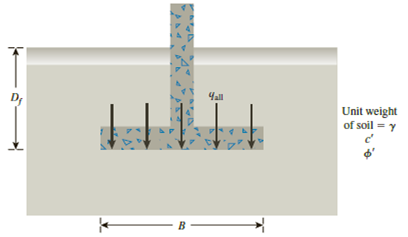# A continuous footing is shown in Figure 16.17. Using Terzaghi’s bearing capacity factors, determine the gross allowable load per unit area ( q all ) that the footing can carry. Assume general shear failure. Given: γ = 19 kN/m 3 , c ′ = 31kN/m 2 , ϕ ′ = 28 ° , D f = 1.5 m, B = 2 m, and factor of safety = 3.5. Figure 16.17### Principles of Geotechnical Enginee...

9th Edition
Braja M. Das + 1 other
Publisher: Cengage Learning
ISBN: 9781305970939### Principles of Geotechnical Enginee...

9th Edition
Braja M. Das + 1 other
Publisher: Cengage Learning
ISBN: 9781305970939

#### Solutions

Chapter
Section
Chapter 16, Problem 16.1P
Textbook Problem

## A continuous footing is shown in Figure 16.17. Using Terzaghi’s bearing capacity factors, determine the gross allowable load per unit area (qall) that the footing can carry. Assume general shear failure. Given: γ = 19 kN/m3, c′ = 31kN/m2, ϕ ′ = 28 ° , Df = 1.5 m, B = 2 m, and factor of safety = 3.5.Figure 16.17

Expert Solution
To determine

Find the gross allowable load per unit area (qall) that the footing can carry.

The gross allowable load per unit area (qall) that the footing can carry is 499.4kN/m2_.

### Explanation of Solution

Given information:

The unit weight of the soil γ is 19kN/m3.

The value of cohesion c is 31kN/m2.

The soil friction angle ϕ is 28°.

The depth of foundation Df is 1.5 m.

The width of footing B is 2 m.

The factor of safety Fs is 3.5.

Calculation:

Determine the ultimate bearing capacity of the soil (qu) using the relation.

qu=cNc+qNq+12γBNγ=cNc+γDfNq+12γBNγ (1)

Here, Nc is the contribution of cohesion, Nq is the contribution of surcharge, and Nγ is the contribution of unit weight of soil.

Refer Table 16.1, “Terzaghi’s bearing-capacity factors–Nc, Nq, and Nγ” in the textbook.

Take Nc as 31.61, Nq as 17.81, and Nγ as 13.70 for the ϕ value of 28°.

Substitute 31kN/m2 for c, 31.61 for Nc, 19kN/m3 for γ, 1.5 m for Df, 17.81 for Nq, 2 m for B, and 13.70 for Nγ in Equation (1).

qu=(31×31.61)+(19×1.5×17.81)+(12×19×2×13.70)=1,747.795kN/m2

Determine the gross allowable load per unit area (qall) that the footing can carry using the relation.

qall=quFs

Substitute 1,747.795kN/m2 for qu and 3.5 for Fs.

qall=1,747.7953.5=499.4kN/m2

Therefore, the gross allowable load per unit area (qall) that the footing can carry is 499.4kN/m2_.

### Want to see more full solutions like this?

Subscribe now to access step-by-step solutions to millions of textbook problems written by subject matter experts!

Get Solutions

### Want to see more full solutions like this?

Subscribe now to access step-by-step solutions to millions of textbook problems written by subject matter experts!

Get Solutions

Find more solutions based on key concepts
Show solutions
For Problems 16.29 through 16.32, show the dimensions of the object on its orthographic views. 16.32 All dimens...

Engineering Fundamentals: An Introduction to Engineering (MindTap Course List)

How could Lewins force field analysis be applied to this project?

Principles of Information Systems (MindTap Course List)

What are the main components of a database system?

Database Systems: Design, Implementation, & Management

What is the primary value of a firewall?

Principles of Information Security (MindTap Course List)

What is a DBMS, and what are its functions?

Database Systems: Design, Implementation, & Management

How would you explode DFDs?

Systems Analysis and Design (Shelly Cashman Series) (MindTap Course List)

Define the term, operating system. List the functions of an operating system.

Enhanced Discovering Computers 2017 (Shelly Cashman Series) (MindTap Course List)

If your motherboard supports ECC DDR3 memory, can you substitute non-ECC DDR3 memory?

A+ Guide to Hardware (Standalone Book) (MindTap Course List)